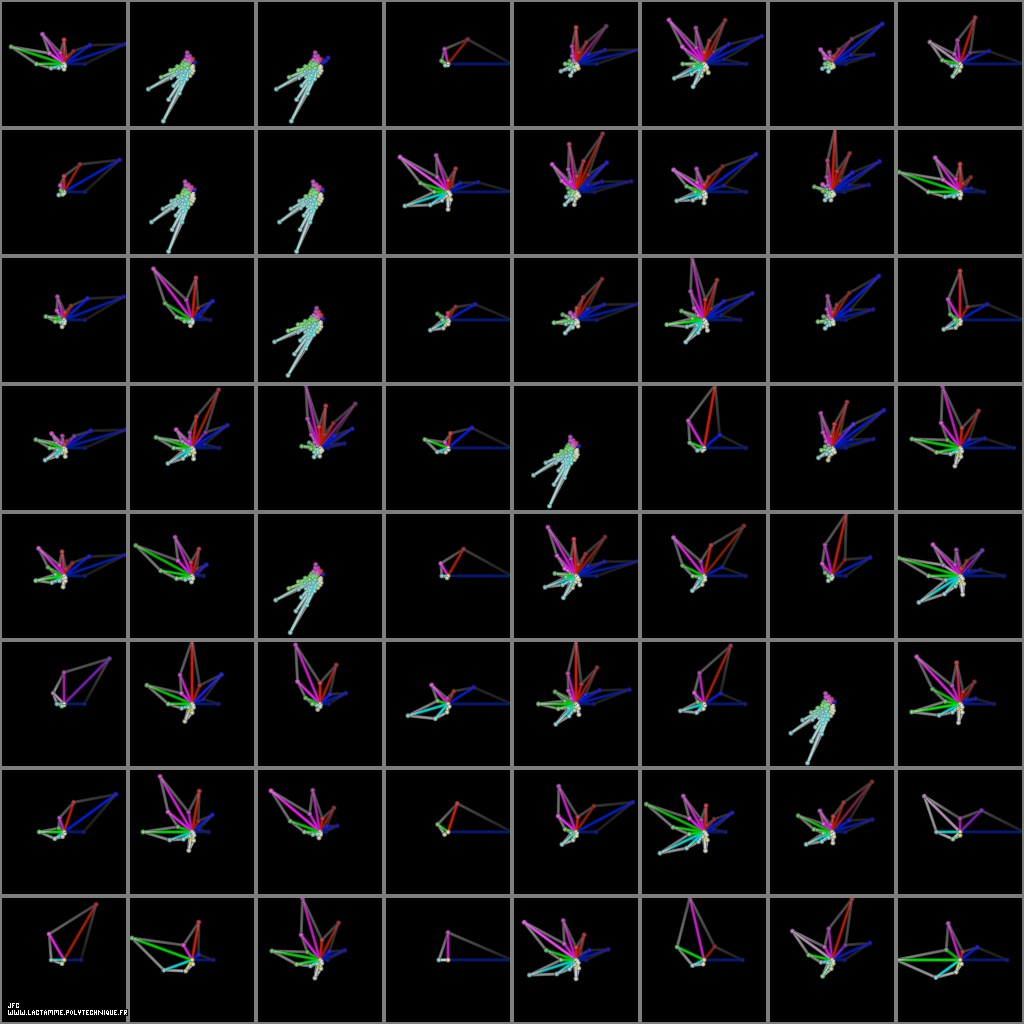The Syracuse conjecture for U(0)={5,6,7,8,...,68} -polar coordinates display- [La conjecture de Syracuse pour U(0)={5,6,7,8,...,68} -visualisation en coordonnées polaires-].

• The Syracuse sequence is defined as follows:

## ``` U = N (an integer number [un nombre entier]) > 0 0 if U is even [si U est pair] : n n U n U = ---- n+1 2 else [sinon] : U = 3*U + 1 n+1 n ```

• The Syracuse conjecture states that sooner or later the {[[4,] 2,] 1} sequence will appear whatever the starting number N (and then repeats itself obviously ad vitam aeternam). For example with U(0)=7:
```                    U(0)  =  7
U(1)  = 22
U(2)  = 11
U(3)  = 34
U(4)  = 17
U(5)  = 52
U(6)  = 26
U(7)  = 13
U(8)  = 40
U(9)  = 20
U(10) = 10
U(11) =  5
U(12) = 16
U(13) =  8
U(14) =  4
U(15) =  2
U(16) =  1
```

Here are 256 different sequences starting from U(0)=1 to U(0)=256.

• This picture is a circular display of sixty-four different sequences from U(0)=5 (lower left) to U(0)=68 (upper right). For each sequence U(n) the following "star" is generated:
```                    Rho(n)  = U  (with a renormalization inside [0,1])
n
```
```                               2.pi
Teta(n) = ------.n
nM+1
```
```                    X(n)    = Rho(n).cos(Teta(n))
Y(n)    = Rho(n).sin(Teta(n))
```
where 'nM' denotes the first 'n' such as:
```                    U   = 1
nM
```
The colors used are a function of 'n' (from Dark Blue [n=0] to White with an increasing luminance).

See some related visualizations (including this one):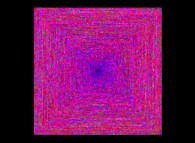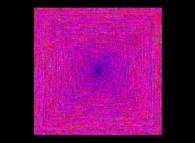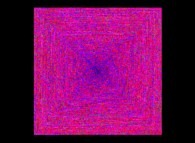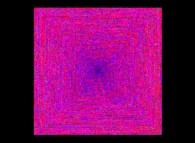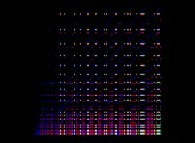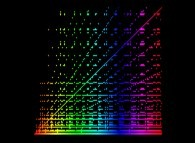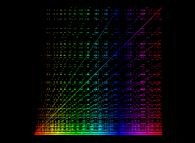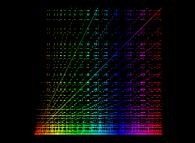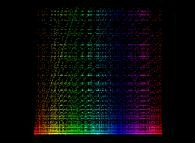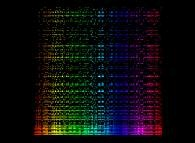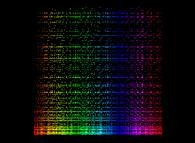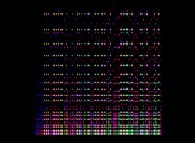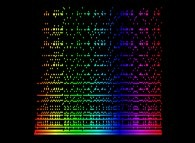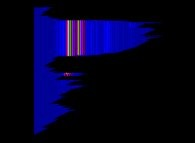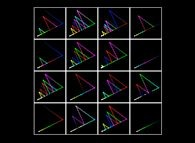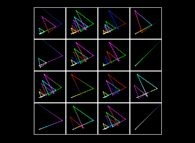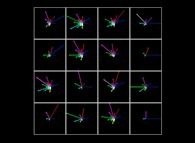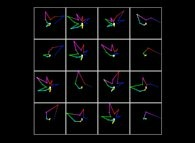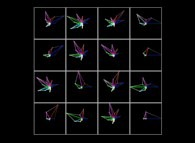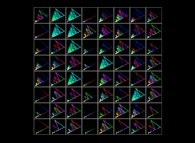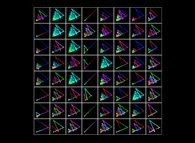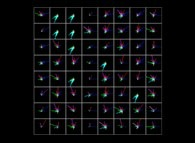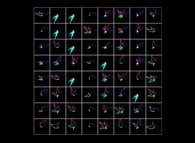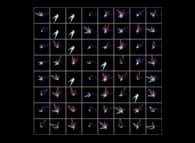(CMAP28 WWW site: this page was created on 01/24/2013 and last updated on 05/06/2020 10:58:25 -CEST-)

[See all related pictures (including this one) [Voir toutes les images associées (incluant celle-ci)]]

[Go back to AVirtualSpaceTimeTravelMachine [Retour à AVirtualSpaceTimeTravelMachine]]
[The Y2K bug [Le bug de l'an 2000]]

[Site Map, Help and Search [Plan du Site, Aide et Recherche]]
[Mail [Courrier]]
[About Pictures and Animations [A Propos des Images et des Animations]]